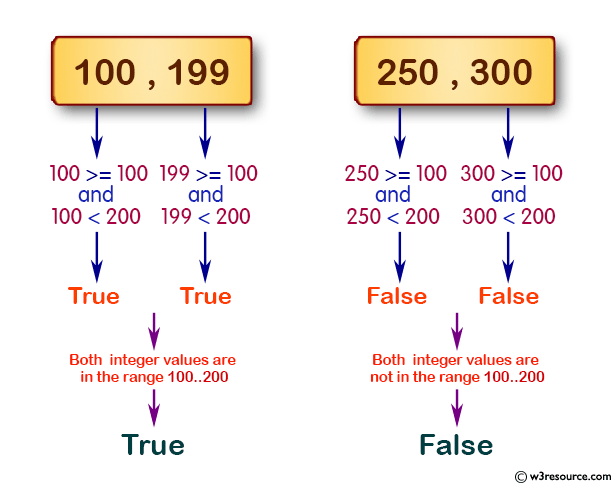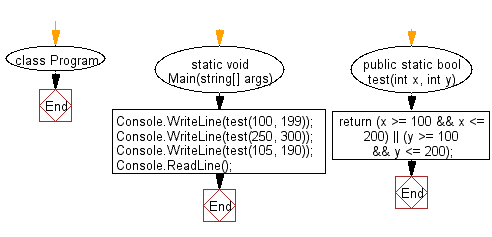﻿ C# - Find out whether two integers are in the range 100..200# C# Sharp Basic Algorithm Exercises: Check two given integers whether either of them is in the range 100..200 inclusive

## C# Sharp Basic Algorithm: Exercise-14 with Solution

Write a C# Sharp program to check two given integers whether either of them is in the range 100..200 inclusive.

Pictorial Presentation:Sample Solution:

C# Sharp Code:

``````using System;

namespace exercises
{
class Program
{
static void Main(string[] args)
{
Console.WriteLine(test(100, 199));
Console.WriteLine(test(250, 300));
Console.WriteLine(test(105, 190));
}
public static bool test(int x, int y)
{
return (x >= 100 && x <= 200) || (y >= 100 && y <= 200);
}
}
}
```
```

Sample Output:

```True
False
True```

Flowchart:C# Sharp Code Editor:

Improve this sample solution and post your code through Disqus

What is the difficulty level of this exercise?

Test your Programming skills with w3resource's quiz.

﻿